Next: Properties of entropy Up: Statistical thermodynamics Previous: General interaction between macrosystems

## Entropy

Consider an isolated system whose energy is known to lie in a narrow range. Let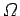be the number of accessible microstates. According to the principle of equal a priori probabilities, the system is equally likely to be found in any one of these states when it is in thermal equilibrium. The accessible states are just that set of microstates which are consistent with the macroscopic constraints imposed on the system. These constraints can usually be quantified by specifying the values of some parameters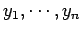which characterize the macrostate. Note that these parameters are not necessarily external: e.g., we could specify either the volume (an external parameter) or the mean pressure (the mean force conjugate to the volume). The number of accessible states is clearly a function of the chosen parameters, so we can write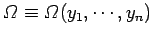for the number of microstates consistent with a macrostate in which the general parameter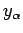lies in the rangeto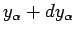.

Suppose that we start from a system in thermal equilibrium. According to statistical mechanics, each of the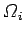, say, accessible states are equally likely. Let us now remove, or relax, some of the constraints imposed on the system. Clearly, all of the microstates formally accessible to the system are still accessible, but many additional states will, in general, become accessible. Thus, removing or relaxing constraints can only have the effect of increasing, or possibly leaving unchanged, the number of microstates accessible to the system. If the final number of accessible states is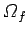, then we can write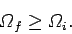(211)

Immediately after the constraints are relaxed, the systems in the ensemble are not in any of the microstates from which they were previously excluded. So the systems only occupy a fraction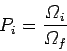(212)

of thestates now accessible to them. This is clearly not a equilibrium situation. Indeed, ifthen the configuration in which the systems are only distributed over the originalstates is an extremely unlikely one. In fact, its probability of occurrence is given by Eq. (212). According to the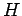theorem (see Sect. 3.4), the ensemble will evolve in time until a more probable final state is reached in which the systems are evenly distributed over theavailable states.

As a simple example, consider a system consisting of a box divided into two regions of equal volume. Suppose that, initially, one region is filled with gas and the other is empty. The constraint imposed on the system is, thus, that the coordinates of all of the molecules must lie within the filled region. In other words, the volume accessible to the system is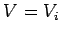, where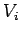is half the volume of the box. The constraints imposed on the system can be relaxed by removing the partition and allowing gas to flow into both regions. The volume accessible to the gas is now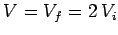. Immediately after the partition is removed, the system is in an extremely improbable state. We know, from Sect. 3.8, that at constant energy the variation of the number of accessible states of an ideal gas with the volume is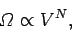(213)

whereis the number of particles. Thus, the probability of observing the state immediately after the partition is removed in an ensemble of equilibrium systems with volume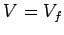is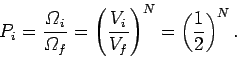(214)

If the box contains of order 1 mole of molecules then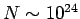and this probability is fantastically small: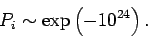(215)

Clearly, the system will evolve towards a more probable state.

This discussion can also be phrased in terms of the parametersof the system. Suppose that a constraint is removed. For instance, one of the parameters,, say, which originally had the value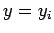, is now allowed to vary. According to statistical mechanics, all states accessible to the system are equally likely. So, the probability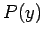of finding the system in equilibrium with the parameter in the rangeto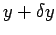is just proportional to the number of microstates in this interval: i.e.,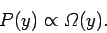(216)

Usually,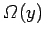has a very pronounced maximum at some particular value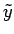(see Sect. 5.2). This means that practically all systems in the final equilibrium ensemble have values ofclose to. Thus, if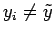initially then the parameterwill change until it attains a final value close to, whereis maximum. This discussion can be summed up in a single phrase:
If some of the constraints of an isolated system are removed then the parameters of the system tend to readjust themselves in such a way that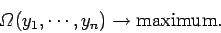(217)

Suppose that the final equilibrium state has been reached, so that the systems in the ensemble are uniformly distributed over theaccessible final states. If the original constraints are reimposed then the systems in the ensemble still occupy thesestates with equal probability. Thus, if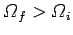, simply restoring the constraints does not restore the initial situation. Once the systems are randomly distributed over thestates they cannot be expected to spontaneously move out of some of these states and occupy a more restricted class of states merely in response to the reimposition of a constraint. The initial condition can also not be restored by removing further constraints. This could only lead to even more states becoming accessible to the system.

Suppose that some process occurs in which an isolated system goes from some initial configuration to some final configuration. If the final configuration is such that the imposition or removal of constraints cannot by itself restore the initial condition then the process is deemed irreversible. On the other hand, if it is such that the imposition or removal of constraints can restore the initial condition then the process is deemed reversible. From what we have already said, an irreversible process is clearly one in which the removal of constraints leads to a situation where. A reversible process corresponds to the special case where the removal of constraints does not change the number of accessible states, so that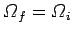. In this situation, the systems remain distributed with equal probability over these states irrespective of whether the constraints are imposed or not.

Our microscopic definition of irreversibility is in accordance with the macroscopic definition discussed in Sect. 3.6. Recall that on a macroscopic level an irreversible process is one which looks unphysical'' when viewed in reverse. On a microscopic level it is clearly plausible that a system should spontaneously evolve from an improbable to a probable configuration in response to the relaxation of some constraint. However, it is quite clearly implausible that a system should ever spontaneously evolve from a probable to an improbable configuration. Let us consider our example again. If a gas is initially restricted to one half of a box, via a partition, then the flow of gas from one side of the box to the other when the partition is removed is an irreversible process. This process is irreversible on a microscopic level because the initial configuration cannot be recovered by simply replacing the partition. It is irreversible on a macroscopic level because it is obviously unphysical for the molecules of a gas to spontaneously distribute themselves in such a manner that they only occupy half of the available volume.

It is actually possible to quantify irreversibility. In other words, in addition to stating that a given process is irreversible, we can also give some indication of how irreversible it is. The parameter which measures irreversibility is just the number of accessible states. Thus, iffor an isolated system spontaneously increases then the process is irreversible, the degree of irreversibility being proportional to the amount of the increase. Ifstays the same then the process is reversible. Of course, it is unphysical forto ever spontaneously decrease. In symbols, we can write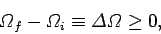(218)

for any physical process operating on an isolated system. In practice,itself is a rather unwieldy parameter with which to measure irreversibility. For instance, in the previous example, where an ideal gas doubles in volume (at constant energy) due to the removal of a partition, the fractional increase inis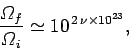(219)

whereis the number of moles. This is an extremely large number! It is far more convenient to measure irreversibility in terms of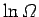. If Eq. (218) is true then it is certainly also true that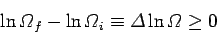(220)

for any physical process operating on an isolated system. The increase inwhen an ideal gas doubles in volume (at constant energy) is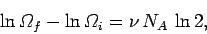(221)

where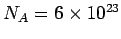. This is a far more manageable number! Since we usually deal with particles by the mole in laboratory physics, it makes sense to pre-multiply our measure of irreversibility by a number of order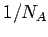. For historical reasons, the number which is generally used for this purpose is the Boltzmann constant, which can be written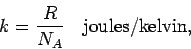(222)

where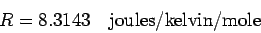(223)

is the ideal gas constant which appears in the well-known equation of state for an ideal gas,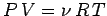. Thus, the final form for our measure of irreversibility is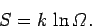(224)

This quantity is termed entropy'', and is measured in joules per degree kelvin. The increase in entropy when an ideal gas doubles in volume (at constant energy) is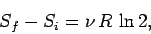(225)

which is order unity for laboratory scale systems (i.e., those containing about one mole of particles). The essential irreversibility of macroscopic phenomena can be summed up as follows: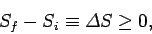(226)

for a process acting on an isolated system [this is equivalent to Eqs. (217), (218), and (220)]. Thus, the entropy of an isolated system tends to increase with time and can never decrease. This proposition is known as the second law of thermodynamics.

One way of thinking of the number of accessible statesis that it is a measure of the disorder associated with a macrostate. For a system exhibiting a high degree of order we would expect a strong correlation between the motions of the individual particles. For instance, in a fluid there might be a strong tendency for the particles to move in one particular direction, giving rise to an ordered flow of the system in that direction. On the other hand, for a system exhibiting a low degree of order we expect far less correlation between the motions of individual particles. It follows that, all other things being equal, an ordered system is more constrained than a disordered system, since the former is excluded from microstates in which there is not a strong correlation between individual particle motions, whereas the latter is not. Another way of saying this is that an ordered system has less accessible microstates than a corresponding disordered system. Thus, entropy is effectively a measure of the disorder in a system (the disorder increases with). With this interpretation, the second law of thermodynamics reduces to the statement that isolated systems tend to become more disordered with time, and can never become more ordered.

Note that the second law of thermodynamics only applies to isolated systems. The entropy of a non-isolated system can decrease. For instance, if a gas expands (at constant energy) to twice its initial volume after the removal of a partition, we can subsequently recompress the gas to its original volume. The energy of the gas will increase because of the work done on it during compression, but if we absorb some heat from the gas then we can restore it to its initial state. Clearly, in restoring the gas to its original state, we have restored its original entropy. This appears to violate the second law of thermodynamics because the entropy should have increased in what is obviously an irreversible process (just try to make a gas spontaneously occupy half of its original volume!). However, if we consider a new system consisting of the gas plus the compression and heat absorption machinery, then it is still true that the entropy of this system (which is assumed to be isolated) must increase in time. Thus, the entropy of the gas is only kept the same at the expense of increasing the entropy of the rest of the system, and the total entropy is increased. If we consider the system of everything in the Universe, which is certainly an isolated system since there is nothing outside it with which it could interact, then the second law of thermodynamics becomes:

The disorder of the Universe tends to increase with time and can never decrease.

An irreversible process is clearly one which increases the disorder of the Universe, whereas a reversible process neither increases nor decreases disorder. This definition is in accordance with our previous definition of an irreversible process as one which does not look right'' when viewed backwards. One easy way of viewing macroscopic events in reverse is to film them, and then play the film backwards through a projector. There is a famous passage in the novel Slaughterhouse 5,'' by Kurt Vonnegut, in which the hero, Billy Pilgrim, views a propaganda film of an American World War II bombing raid on a German city in reverse. This is what the film appeared to show:

American planes, full of holes and wounded men and corpses took off backwards from an airfield in England. Over France, a few German fighter planes flew at them backwards, sucked bullets and shell fragments from some of the planes and crewmen. They did the same for wrecked American bombers on the ground, and those planes flew up backwards and joined the formation.

The formation flew backwards over a German city that was in flames. The bombers opened their bomb bay doors, exerted a miraculous magnetism which shrunk the fires, gathered them into cylindrical steel containers, and lifted the containers into the bellies of the planes. The containers were stored neatly in racks. The Germans had miraculous devices of their own, which were long steel tubes. They used them to suck more fragments from the crewmen and planes. But there were still a few wounded Americans, though, and some of the bombers were in bad repair. Over France, though, German fighters came up again, made everything and everybody as good as new.''
Vonnegut's point, I suppose, is that the morality of actions is inverted when you view them in reverse.

What is there about this passage which strikes us as surreal and fantastic? What is there that immediately tells us that the events shown in the film could never happen in reality? It is not so much that the planes appear to fly backwards and the bombs appear to fall upwards. After all, given a little ingenuity and a sufficiently good pilot, it is probably possible to fly a plane backwards. Likewise, if we were to throw a bomb up in the air with just the right velocity we could, in principle, fix it so that the velocity of the bomb matched that of a passing bomber when their paths intersected. Certainly, if you had never seen a plane before it would not be obvious which way around it was supposed to fly. However, certain events are depicted in the film, miraculous'' events in Vonnegut's words, which would immediately strike us as the wrong way around even if we had never seen them before. For instance, the film might show thousands of white hot bits of shrapnel approach each other from all directions at great velocity, compressing an explosive gas in the process, which slows them down such that when they meet they fit together exactly to form a metal cylinder enclosing the gases and moving upwards at great velocity. What strikes us as completely implausible about this event is the spontaneous transition from the disordered motion of the gases and metal fragments to the ordered upward motion of the bomb.Next: Properties of entropy Up: Statistical thermodynamics Previous: General interaction between macrosystems
Richard Fitzpatrick 2006-02-02